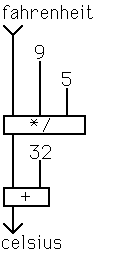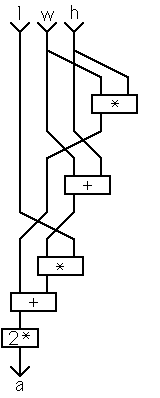# Extending the Dictionary

So far we have seen how to use the command line interpreter, and some of the words in the Forth dictionary. Here we will see one way of adding new words to the dictionary.

In addition to the sorts of words that we have seen so far Forth comes with a selection of simple compilers that allow new words to be added to the dictionary.

The most general of these is ":", the "colon compiler".Going back to our previous example, of converting degrees Fahrenheit to degrees Celsius, which, as you recall, was
`     100 9 5 */ 32 + . [cr] 212 ok`
A colon definition to do the conversion would be
`     : F>C ( fahrenheit--celsius)  9 5 */  32 + ;`
Once this is entered Forth knows a new word called F>C.

The first thing we should do is test it, thus;

`     100 F>C . [cr] 212 ok`
Now that we know it works we can use it like any other Forth word.A more complicated problem is finding the surface area (a) of a cuboid, given its length (l), width (w) and height (h).

A moments thought shows that the formula 2(l.w+l.h+w.h) can be rewritten as 2(l.(w+h)+w.h), which has one less multiplication in it.

With a little experience the task of converting this into a colon definition is quite straight forward. The definition given here introduces some new stack operators. Their function should be apparent from the StackFlow diagram.

```
: SURFACE.AREA ( l w h--a)
2DUP *  -ROT +  ROT * + 2* ;
```
As always, the first thing we do having defined a word is test it, so;
`   3 4 5 SURFACE.AREA . [cr] 94 ok`
This is the correct result, so we can accept it. (In real life testing would be more extensive of course.)
Go on to the next section. Go to the contents page.